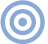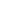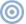# Reference

## The Jitter Game The Timing Problem

Sidebar 1: The Timing Problem

For the mathematically inclined, the signal/noise ratio (SNR) in a digital system is affected by time-base jitter in the following manner:

SNR = 20 log10(2 T PI F Square Root(2 F0/FS))

where: T = RMS jitter PI = 3.1416 F = signal frequency F0 = bandwidth of noise measurement (20kHz) FS = sampling frequency (352,800Hz for 8x oversampling)—Robert HarleyARTICLE CONTENTSX